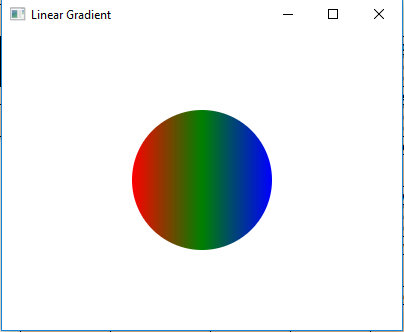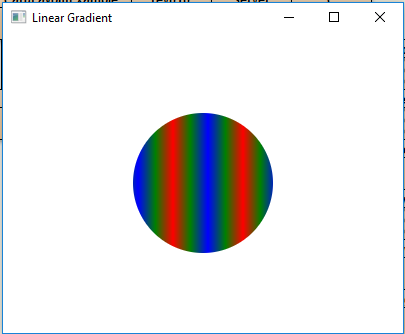LinearGradient class is a part of JavaFX. LinearGradient class fills a shape with a linear color gradient pattern. A user may specify more than one LinearGradient pattern and the system will provide interpolation between the colors.

Constructors of the class:

1. LinearGradient(double sX, double sY, double eX, double eY, boolean prop, CycleMethod c, List s): Creates a new Lineargradient object.
2. LinearGradient(double sX, double sY, double eX, double eY, boolean prop, CycleMethod c, Stop… s): Creates a new LinearGradient object.

Commonly Used Methods:

Method Explanation
equals(Object o) Returns whether the LinearGradient objects are equal or not.
getCycleMethod() Returns the cycle method of the linear gradient object.
getEndX() Returns the x coordinate of end point of the linear gradient.
getEndY() Returns the y coordinate of end point of the linear gradient.
getStartX() Returns the x coordinate of start point of the linear gradient.
getStartY() Returns the y coordinate of start point of the linear gradient.
getStops() Returns the stops of linear gradient.
isOpaque() Returns whether the linear Gradient is opaque or not.
isProportional() Returns whether the linear Gradient is proportional or not.
valueOf(String v) Creates a linear gradient value from a string representation.

Below programs illustrate the use of LinearGradient Class:

1. Java program to create a LinearGradient object and add stops to it and apply it to the circle: In this program we will create an array of Stop objects with their offset values ranging from 0 to 1. Create a LinearGradient object with specified stops. Now create a circle with specified x, y positions, and radius and add the linear gradient to it. Then create a VBox and set the alignment of it. Add the circle to the vbox and add the vbox to the scene and add the scene to the stage and call the show() function to display the results.

 `// Java program to create a LinearGradient  ` `// object and add stops to it and apply it ` `// to the circle ` `import` `javafx.application.Application; ` `import` `javafx.scene.Scene; ` `import` `javafx.scene.control.*; ` `import` `javafx.scene.layout.*; ` `import` `javafx.stage.Stage; ` `import` `javafx.scene.layout.*; ` `import` `javafx.scene.paint.*; ` `import` `javafx.scene.text.*; ` `import` `javafx.geometry.*; ` `import` `javafx.scene.layout.*; ` `import` `javafx.scene.shape.*; ` `import` `javafx.scene.paint.*; ` `  `  `public` `class` `Linear_Gradient_1 ``extends` `Application { ` `  `  `// launch the application ` `public` `void` `start(Stage stage) ` `{ ` ` `  `    ``try` `{ ` ` `  `        ``// set title for the stage ` `        ``stage.setTitle(``"Linear Gradient"``); ` ` `  `        ``// create stops ` `        ``Stop[] stop = {``new` `Stop(``0``, Color.RED),  ` `                       ``new` `Stop(``0.5``, Color.GREEN),  ` `                       ``new` `Stop(``1``, Color.BLUE)}; ` ` `  `        ``// create a Linear gradient object ` `        ``LinearGradient linear_gradient = ``new` `LinearGradient(``0``, ``0``, ` `                          ``1``, ``0``, ``true``, CycleMethod.NO_CYCLE, stop); ` ` `  `        ``// create a circle ` `        ``Circle circle = ``new` `Circle(``100``, ``100``, ``70``); ` ` `  `        ``// set fill ` `        ``circle.setFill(linear_gradient); ` ` `  `        ``// create VBox ` `        ``VBox vbox = ``new` `VBox(circle); ` ` `  `        ``// ste Alignment ` `        ``vbox.setAlignment(Pos.CENTER); ` ` `  `        ``// create a scene ` `        ``Scene scene = ``new` `Scene(vbox, ``400``, ``300``); ` ` `  `        ``// set the scene ` `        ``stage.setScene(scene); ` ` `  `        ``stage.show(); ` `    ``} ` ` `  `    ``catch` `(Exception e) { ` ` `  `        ``System.out.println(e.getMessage()); ` `    ``} ` `} ` ` `  `// Main Method ` `public` `static` `void` `main(String args[]) ` `{ ` ` `  `    ``// launch the application ` `    ``launch(args); ` `} ` `} `

Output:2. Java program to create a LinearGradient object and add stops to it and set the CycleMethod to reflect and set proportional to false and apply it to the circle: In this program we will create an array of Stop objects with their offset values ranging from 0 to 1. Then create a LinearGradient object with specified stops. Set the CycleMethod to reflect and set proportional to false. Create a circle with specified x, y positions, and radius and add the linear gradient to it. Then create a VBox and set the alignment of it. Add the circle to the vbox and add the vbox to the scene and add the scene to the stage and call the show() function to display the results.

 `// Java program to create a LinearGradient object  ` `// and add stops to it and set the CycleMethod to ` `// reflect and set proportional to false and  ` `// apply it to the circle ` `import` `javafx.application.Application; ` `import` `javafx.scene.Scene; ` `import` `javafx.scene.control.*; ` `import` `javafx.scene.layout.*; ` `import` `javafx.stage.Stage; ` `import` `javafx.scene.layout.*; ` `import` `javafx.scene.paint.*; ` `import` `javafx.scene.text.*; ` `import` `javafx.geometry.*; ` `import` `javafx.scene.layout.*; ` `import` `javafx.scene.shape.*; ` `import` `javafx.scene.paint.*; ` ` `  `public` `class` `Linear_Gradient_2 ``extends` `Application { ` ` `  `    ``// launch the application ` `    ``public` `void` `start(Stage stage) ` `    ``{ ` ` `  `        ``try` `{ ` ` `  `            ``// set title for the stage ` `            ``stage.setTitle(``"Linear Gradient"``); ` ` `  `            ``// create stops ` `            ``Stop[] stop = {``new` `Stop(``0``, Color.RED), ``new` `Stop(``0.5``,  ` `                         ``Color.GREEN), ``new` `Stop(``1``, Color.BLUE)}; ` ` `  `            ``// create a Linear gradient object ` `            ``LinearGradient linear_gradient = ``new` `LinearGradient(``0``, ``0``,  ` `                            ``35``, ``0``, ``false``, CycleMethod.REFLECT, stop); ` ` `  `            ``// create a circle ` `            ``Circle circle = ``new` `Circle(``100``, ``100``, ``70``); ` ` `  `            ``// set fill ` `            ``circle.setFill(linear_gradient); ` ` `  `            ``// create VBox ` `            ``VBox vbox = ``new` `VBox(circle); ` ` `  `            ``// ste Alignment ` `            ``vbox.setAlignment(Pos.CENTER); ` ` `  `            ``// create a scene ` `            ``Scene scene = ``new` `Scene(vbox, ``400``, ``300``); ` ` `  `            ``// set the scene ` `            ``stage.setScene(scene); ` ` `  `            ``stage.show(); ` `        ``} ` ` `  `        ``catch` `(Exception e) { ` ` `  `            ``System.out.println(e.getMessage()); ` `        ``} ` `    ``} ` ` `  `    ``// Main Method ` `    ``public` `static` `void` `main(String args[]) ` `    ``{ ` ` `  `        ``// launch the application ` `        ``launch(args); ` `    ``} ` `} `

Output:Note: The above programs might not run in an online IDE please use an offline compiler.

Attention reader! Don’t stop learning now. Get hold of all the important DSA concepts with the DSA Self Paced Course at a student-friendly price and become industry ready.

My Personal Notes arrow_drop_upCheck out this Author's contributed articles.

If you like GeeksforGeeks and would like to contribute, you can also write an article using contribute.geeksforgeeks.org or mail your article to contribute@geeksforgeeks.org. See your article appearing on the GeeksforGeeks main page and help other Geeks.

Please Improve this article if you find anything incorrect by clicking on the "Improve Article" button below.

Article Tags :
Practice Tags :

Be the First to upvote.

Please write to us at contribute@geeksforgeeks.org to report any issue with the above content.